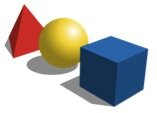# ProbabilityWhat are the chances that you can flip heads or tails? Probability can be a fun guessing game and is an interactive Math concept that can be simple to introduce to elementary school students. Probability can tell you how likely or unlikely an outcome is, which can give you mathematical information to help you make a decision. If you have a 0.00001% chance of finding gold in your backyard, are you going to start digging for gold? (Shapes; downloaded Feb. 7, 2014;  http://commons.wikimedia.org/wiki/File:Basic_shapes.svg)  Probably not, right? Students can begin to learn that outcomes can be numerically calculated and then can convey valuable information.

In this activity students will learn how to calculate and interpret probability.

Materials:

Large bag of assorted color candy

Small plastic bowls

*It is best if you know how many color of each candy is present in the bag. If this is not possible, estimate the approx. numbers of each.

Instructions

1. Assemble the class into small groups.
2. Give each group a small plastic bowl and 1-2 cups of candy in each group’s bowl.
3. Have the students count the total number of candies, the number of candies of each color and record the data on a piece of paper.
4. Explain to the students that the probability of something occurring is another way of saying what are the chances or the likelihood that an event will happen. You may consider saying, “We are going to calculate the probability of you getting a red colored candy from your bowl, if you picked the candy with your eyes closed. The probability is a number that helps you determine if something is likely to happen or not likely to happen. To find the probability of picking a red candy, we need two numbers. The number of red candies in your bowl and the total number of candies in the bowl. In this group, they have 2 red candies and 31 total candies. So the probability would be 2/31, which is a very small number. So, if they were to close their eyes and choose a candy, it would most likely not be red.”
5. Invite students to calculate the probability of picking each color and allow time for them to pick candies at random to explore the concept of probability further.

For older students, extend the activity by practicing how to reduce fractions, for example a probability of 3/18 can be reduced to 1/6. There are lots of fun ways to teach probability. Check out these great online resources:  http://nrich.maths.org/probability and http://www.scholastic.com/browse/article.jsp?id=3756484.

For more fun and interesting Learning Math Games, you can visit us here:
http://www.math-lessons.ca/activities/FractionsBoard5.html
http://www.math-lessons.ca/timestables/times-tables.html
http://www.math-lessons.ca/activities/FractionsBoard4.html